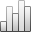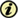Acta Stereologica

0351-580XMore Statisticssince 05 December 2013 :
View(s): 241 (1 ULiège)
Wang Meiqiang, Ulrik Skands & Knut Conradsen

### Evaluation of fracture roughness using two kinds of fractal dimension measurements

Article#### Abstract

This paper introduces the basics of fractal measurements. The fracture surface roughness and the toughness of a series of steel samples were analyzed using two kinds of fractal dimensions.

Fracture surface profiles were obtained by means of the cross vertical sections through the fracture surface of steel samples coated with copper in order to protect the surface. With image processing, the fractal dimension of numerous profiles was measured by Euclidean erosion and dilation through the distance map. This 1D fractal dimension measure described the roughness of fracture surfaces and was correlated with the fracture toughness of the materials.

We also applied mathematical morphology fractals to analyze the grey level images of fracture surfaces of steel samples examined by SEM, which were treated as three dimensional surfaces. The grey level morphological method was based on a series of dilations on this surface and plotting area of the resulting set of surfaces versus the structuring element size. It was demonstrated that the 2D fractal dimension measure was strongly correlated with both rouglmess and fracture toughness.

Keywords : fractal dimension, Fracture, Roughness, toughness

Wang Meiqiang, Ulrik Skands & Knut Conradsen, «Evaluation of fracture roughness using two kinds of fractal dimension measurements», Acta Stereologica [En ligne], Volume 14 (1995), Number 1 - Stermat '94 - Sep. 1995, 45-50 URL : https://popups.ulg.ac.be/0351-580x/index.php?id=673.

#### About: Wang Meiqiang

Institute of Mathematical Modelling, Building 321, Technical University of Denmark, DK-2800 Lyngby, Denmark

#### About: Ulrik Skands

Institute of Mathematical Modelling, Building 321, Technical University of Denmark, DK-2800 Lyngby, Denmark